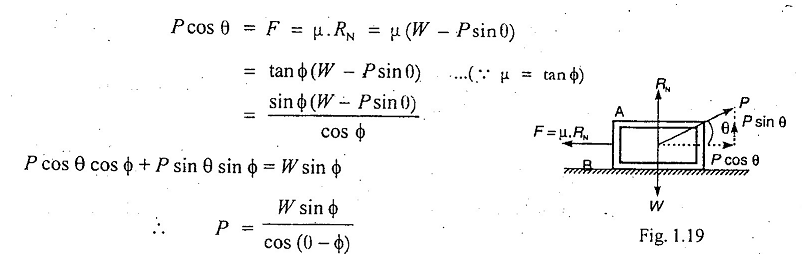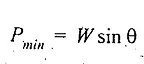# Minimum Force Required to Slide a Body on Horizontal Plane

Minimum Force Required to Slide a Body on a Rough Horizontal Plane

Let a body A of weight W is resting on a rough horizontal plane B, as shown in Fig. 1.19. Let an effort P is applied at an angle θ to the horizontal, such that the body just begins to move. From Fig. 1.19, we find thatFor P to be minimum, cos (θ - ϕ) should be maximum, i.e.

cos (θ-ϕ)=1 or θ-ϕ=0 or θ=0

In other words, the effort P is minimum when its inclination (θ) with the horizontal is equal to the angle of friction (ϕ).### You may like these posts:

1.2.3.